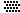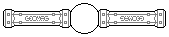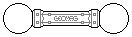Web textodigital.com
Construction of the reinforced rhombicosidodecahedron, method 2
We explain in this page an alternative construction method for the same reinforcing internal structure which was explained in Construction of the reinforced rhombicosidodecahedron, method 1. With that method, the structure is stabilized with thirty six square panels: six for the central cube, and thirty for the square faces of the rhombicosidodecahedron.
In this case, we are going to stabilize the same structure in a completely different way, which, in turn, brings a construction method which is also different. We will not use any square now, but twenty-four pentagons, all of them placed inside the object. So, there will not be any panel at the surface, that is, all of its faces will be hollow.
The internal structure is made of six pieces like that shown in the following picture (Johnson solid number 91, called bilunabirotunda), each one of them stabilized with four pentagons. Neither square (the silver, upper one, or the lower, green one) need stabilization with a panel, as they will be rigid when construction is completed.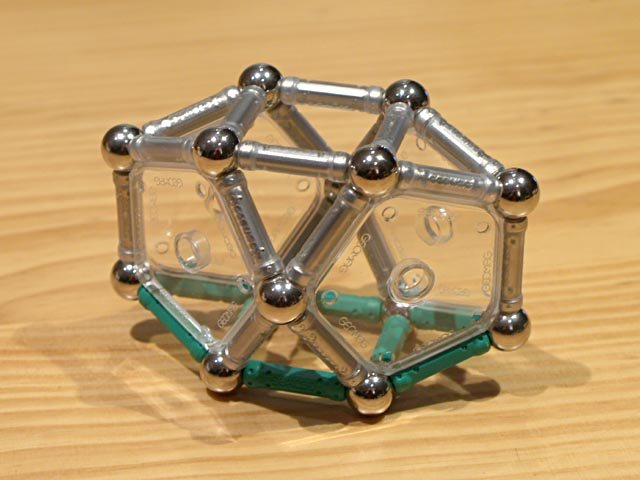Construction of the reinforced rhombicosidodecahedron, method 2, step 1
The green rods are part of the object's surface. That is, the lower green square and two triangles will be faces of the rhombicosidodecahedron, while the upper silver square and triangles, symmetric to the green ones, are part of the auxiliary structure. The square will be a face in the central cube, and the vertex not shared with the square in each triangle will be the apex in an auxiliary pentagonal pyramid.
All six pieces are assembled in parallel pairs, each pair being perpendicular to the others, such as shown in the following pictures, with three, four and all six pieces assembled:Construction of the reinforced rhombicosidodecahedron, method 2, step 2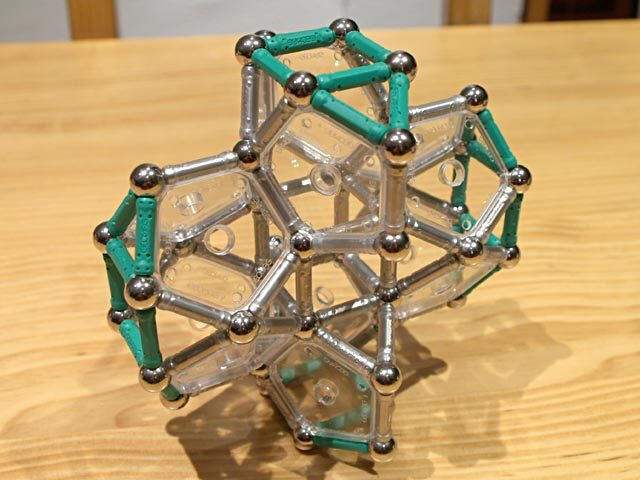Construction of the reinforced rhombicosidodecahedron, method 2, step 3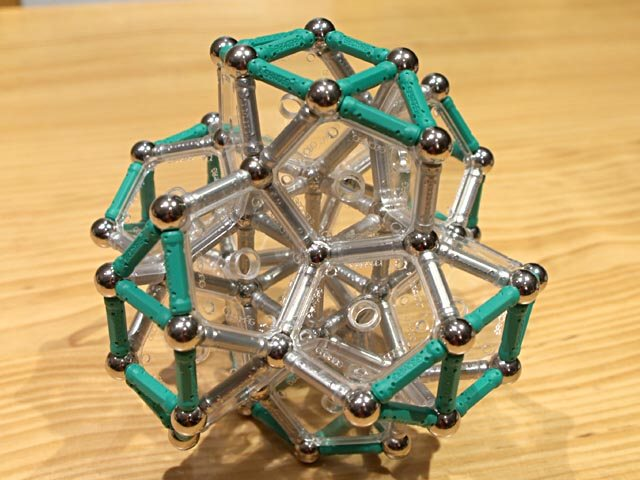Construction of the reinforced rhombicosidodecahedron, method 2, step 4
For each one of the eight trihedral angles in which the pentagons meet, as seen in the previous picture, we add nine rods now, placed as shown in the following picture:Construction of the reinforced rhombicosidodecahedron, method 2, step 5
This makes up all the remaining faces. Only, two auxiliary rods must be added to each pentagonal pyramid (not yet done to the foreground pyramid in this picture, although the quantity of rods given is the total required):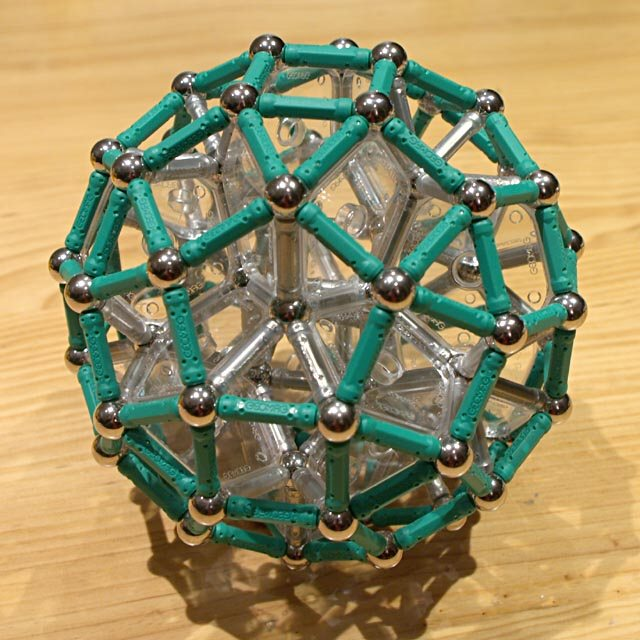Reinforced rhombicosidodecahedron, method 2 320 pieces: 80 balls, 216 rods, 24 pentagons (1.78 kg)